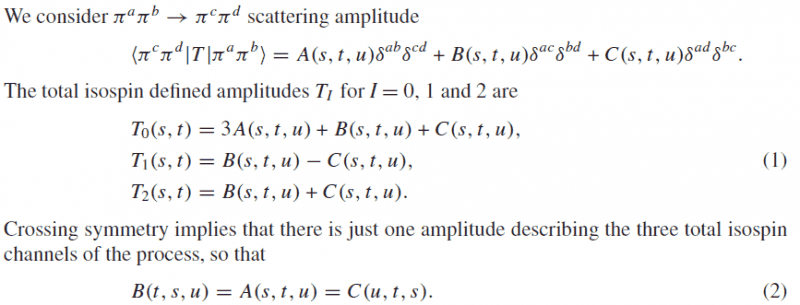# Decomposed isospinHow to use the standard techniques of projection operators to obtain the equation (1) by the first formula? Thanks

vanhees71
Gold Member
2021 Award
Isospin is (approximately) conserved in the strong interaction. Thus you can decompose the total isospin of the two-pion system in irreducible parts. Pions are pseudoscalar mesons with isospin 1 (forming a triplet, ##\pi^+##, ##\pi^-##, and ##\pi^0##). From the theory of angular momentum, i.e., the representation theory of the Lie algebra of su(2), i.e., the commutation rules for angular momenta you know that the direct product of two spin-1 representations splits into the direct sum of the trivial, the ##s=1##, and ##s=2## representation: ##1 \otimes 1=0 \oplus 1 \oplus 2##.

In this case it's simpler to work with a real representation of the pion fields ##\vec{\pi} \in \mathbb{R}^3##. Then the isospin rotations are simply described by real ##\mathrm{SO}(3)## matrices. The split into irreducible parts of the tensor product is then straight forward.

The ##s=0## part of the product of two pion fields is found by looking for a scalar built as a bilinear form of ##\vec{\pi}##. That's uniquely given by the scalar product ##\vec{\pi} \cdot \vec{\pi}=\delta_{ab} \pi^a \pi^b##. To find the corresponding amplitude for this channel thus you simply trace over the index pair ##(a,b)##
$$\delta_{ab} T^{abcd}=(3A+B+C) \delta^{cd}.$$
This gives you the first equation in (1).

The ##s=1## piece is given by the vector product ##\epsilon_{abe} \pi^a \pi^b##. Thus you have to contract the amplitude with ##\epsilon_{abe}## leading to
$$\epsilon_{abd} T^{abcd}=B \epsilon_{cde}+C \epsilon_{dce}=(B-C) \epsilon_{cde},$$
giving you the 2nd line of Eq. (1).

Finally the ##s=2## part is represented by the traceless symmetric part of the tensor ##\vec{\pi} \otimes \vec{\pi}##, i.e., ##3 \pi^a \pi^b -\vec{\pi}^2 \delta^{ab}##. So you just have to symmetrize your amplitude wrt. the index pair ##(a,b)## and subtract the trace, leading to
$$T^{(ab)cd}=2A \delta^{ab} \delta^{cd}+ (C+D) \delta^{ac} \delta^{bd}$$
The trace is
$$\delta_{ab} T^{(ab)cd}=(6A+C+D)\delta_{cd}$$
and thus you get
$$T^{(ab)cd}-\frac{1}{3} (6A+C+D) \delta^{ab} \delta^{cd} = \frac{2}{3} (C+D) \delta^{ac} \delta^{bd},$$
which gives you the corresponding amplitude in the 3rd line of Eq. (1).

Thank you so much!
I am a newcomer in this field, so there are many definitions and notions I cannot catch up. Firstly I still cannot understand how to get the tensor $$3\pi^a\pi^b-\vec{\pi}^2\delta^{ab}$$ and find the way that symmetric amplitude subtracts its trace? Second problem is the symmetric amplitude
$$T^{(ab)cd}=\frac{1}{2}(A\delta^{ab}\delta^{cd}+B\delta^{ac}\delta^{bd}+C\delta^{ad}\delta^{bc}+A\delta^{ba}\delta^{cd}+B\delta^{bc}\delta^{ad}+C\delta^{bd}\delta^{ac})$$.
Could you point out where is my error, because the definition I remembered is not the same as yours. Last but not least, $$2A\delta^{ab}\delta^{cd}+(B+C)\delta^{ac}\delta^{bd}-\frac{1}{3}(6A+B+C)\delta^{ab}\delta^{cd}=(B+C)\delta^{ac}\delta^{bd}-\frac{1}{3}(B+C)\delta^{ab}\delta^{cd}$$, then how to deal with the different between $$\delta^{ab}\delta^{cd}~and~\delta^{ac}\delta^{bd}$$ and the factor $$\frac{2}{3}$$

ChrisVer
Gold Member
How to get that $3 \pi^a \pi^b - \delta^{ab} \pi^2$ is seen by the fact that this combination is the only traceless : (a=b and sum) $3 \pi^a \pi^a - \delta^{aa} \pi^2 = 3 \pi^2 - 3 \pi^2 =0$ and symmetric: (interchanging a,b you get the same result)...
In general these type of combinations can be easier obtained (I think) from Young Tableaux...

For the other question, you just have:
$(C+D) \delta^{ac} \delta^{bd} - \frac{1}{3} (C+D) \delta^{ab} \delta^{cd}$
$\frac{1}{3}(C+D) [3\delta^{ac} \delta^{bd} - \delta^{ab} \delta^{cd} ] =\frac{1}{3}(C+D) [2\delta^{ac} \delta^{bd} + \delta^{ac} \delta^{bd} - \delta^{ab} \delta^{cd} ]$

The last two terms in the brackets cancel out....

How to get that $3 \pi^a \pi^b - \delta^{ab} \pi^2$ is seen by the fact that this combination is the only traceless : (a=b and sum) $3 \pi^a \pi^a - \delta^{aa} \pi^2 = 3 \pi^2 - 3 \pi^2 =0$ and symmetric: (interchanging a,b you get the same result)...
In general these type of combinations can be easier obtained (I think) from Young Tableaux...

For the other question, you just have:
$(C+D) \delta^{ac} \delta^{bd} - \frac{1}{3} (C+D) \delta^{ab} \delta^{cd}$
$\frac{1}{3}(C+D) [3\delta^{ac} \delta^{bd} - \delta^{ab} \delta^{cd} ] =\frac{1}{3}(C+D) [2\delta^{ac} \delta^{bd} + \delta^{ac} \delta^{bd} - \delta^{ab} \delta^{cd} ]$

The last two terms in the brackets cancel out....

Thank you for your reply. The traceless and symmetric for the tensor like the Young using in meson decomposed into octet and decuplet, right?
Well, why the procedure that symmetrizing amplitude with subtracting its trace can stand for T2(s,t), <I=0,I3=0|Tabcd|I=0,I3=0>?(of course, I can get the calculation in isospin=0,1,but I still cannot understand the reasons). And why you can cancel out the $\delta^{ac} \delta^{bd}-\delta^{ab} \delta^{cd} ]$? Is the reason of symmetric in isospin=2, so they are equal to each other?

ChrisVer
Gold Member
I didn't understand the first questions.....
the cancelation of deltas you give are a general feature and doesn't apply for a given representation...
A fast way is to use mathematica to show you that the combination is zero for all a,b,c,d which was the way I used to give a fast answer... As for how to see that, well you could play with symmetries? or try to put some given values on a,b,c,d.... ?

vanhees71
Gold Member
2021 Award
How to derive these "projections" is not such a simple thing, because you need representation theory of the rotation group. A great book about this is Lipkin's "Lie groups for pedestrians".

How to derive these "projections" is not such a simple thing, because you need representation theory of the rotation group. A great book about this is Lipkin's "Lie groups for pedestrians".

Thank you for your recommendation. By the way , $T^{I=2}(s,t)=<I=2,I_3=0|T^{abcd}|I=2,I_3=0>$? I and I3 are isospin and component of isospin for initial final state,respectively

I didn't understand the first questions.....
the cancelation of deltas you give are a general feature and doesn't apply for a given representation...
A fast way is to use mathematica to show you that the combination is zero for all a,b,c,d which was the way I used to give a fast answer... As for how to see that, well you could play with symmetries? or try to put some given values on a,b,c,d.... ?

Thanks again firstly.
In general, $\delta^{ac}\delta^{bd}-\delta^{ab}\delta^{cd}=\varepsilon_{dae}\varepsilon_{bce}\neq 0$, and a,b,c,d,e=1,2,3.
Only when a=d or c=b are above deltas equal to 0.

vanhees71
Thank you for your recommendation. By the way , $T^{I=2}(s,t)=<I=2,I_3=0|T^{abcd}|I=2,I_3=0>$? I and I3 are isospin and component of isospin for initial final state,respectively